Linear Regression with Biological Data
(or the realities of working with real-life data)

Data:  The following data shows the relationship between chirps per second of a striped ground cricket and the corresponding ground temperature.

NOTE: There seems to be some confusion relating to the "units" used in Pierce's data. According to the text, The Song of Insects by George W. Pierce, 1948, page 20, the information and unit labeling, as stated at this site, correctly reflects his findings.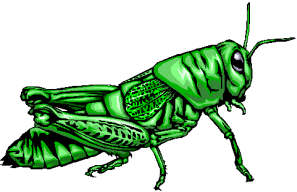Pierce (1948) mechanically measured the frequency (the number of wing vibrations per second) of chirps (or pulses of sound) made by a striped ground cricket, at various ground temperatures.  Since crickets are ectotherms (cold-blooded), the rate of their physiological processes and their overall metabolism are influenced by temperature.  Consequently, there is reason to believe that temperature would have a profound effect on aspects of their behavior, such as chirp frequency. In general, it was found that crickets did not sing at temperatures colder than 60º F. or warmer than 100º F.
 Chirps/Second Temperature (º F) 20.0 88.6 16.0 71.6 19.8 93.3 18.4 84.3 17.1 80.6 15.5 75.2 14.7 69.7 15.7 71.6 15.4 69.4 16.3 83.3 15.0 79.6 17.2 82.6 16.0 80.6 17.0 83.5 14.4 76.3
 Task: a.) Determine a linear regression model equation to represent this data. b.) Graph the new equation. c.) Decide whether the new equation is a "good fit" to represent this data. d.) Extrapolate data:  If the ground temperature reached 95º, then at what approximate rate would you expect the crickets to be chirping? e.) Interpolate data:  With a listening device, you discovered that on a particular morning the crickets were chirping at a rate of 18 chirps per second.  What was the approximate ground temperature that morning? f.) If the ground temperature should drop to freezing (32º F), what happens to the cricket's chirping rate? Answers in this problem are to be rounded to the nearest hundredth.
Step 1.  Enter the data into the lists.
For basic entry of data, see Basic Commands.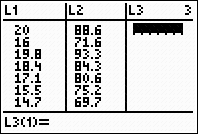Step 2.  Create a scatter plot of the data.
Go to STATPLOT (2nd Y=) and choose the first plot.  Turn the plot ON, set the icon to Scatter Plot (the first one), set Xlist to L1 and Ylist to L2 (assuming that is where you stored the data), and select a Mark of your choice.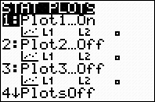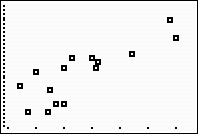Obviously, there is some scatter to this data. This variability is the norm, rather than the exception, when working with biological data sets.  Real life data seldom creates a nice straight line.

Step 3.  Choose the Linear Regression Model.
Press STAT, arrow right to CALC, and arrow down to 4: LinReg (ax+b).  Hit ENTER.  When LinReg appears on the home screen, type the parameters L1, L2, Y1.  The Y1 will put the equation in to Y= for you.
(Y1 comes from VARS → YVARS, #Function, Y1)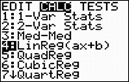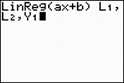Older OS form.Newer OS form.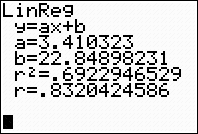The linear regression equation is
y = 3.41x + 22.85

Step 4.  Graph the Linear Regression Equation from Y1.
ZOOM #9 ZoomStat to see the graph.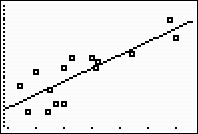Step 5.  Is this model a "good fit"?
The correlation coefficient, r, is .8320424586 which just barely places the correlation into the "strong" category.  (0.8 or greater is a "strong" correlation)
The coefficient of determination, r 2, is .6922946529 which means that 70% of the total variation in y can be explained by the relationship between x and y.  The other 30% remains unexplained.
Yes, it is somewhat of a "good fit".Step 6.  Extrapolate:  (beyond the data set)

If the ground temperature reached 95º, then at what approximate rate would you expect the crickets to be chirping?

Go to TBLSET (above WINDOW) and set the TblStart to 20+ (since the highest temperature in the data set had 20 chirps/second).  Set the delta Tbl to a decimal setting of your choice.  Go to TABLE (above GRAPH) and arrow up or down to find your desired temperature, 95º, in the Y1 column.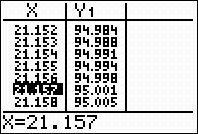(answer to part d --  approx. 21.16 chirps/second)

Step 7.  Interpolate: (within the data set)
With a listening device, you discovered that on a particular morning the crickets were chirping at a rate of 18 chirps per second.  What was the approximate ground temperature that morning?

From the graph screen, hit TRACE, arrow up to obtain the power equation, type 18, hit ENTER, and the answer will appear at the bottom of the screen.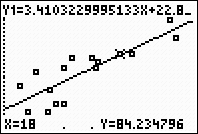(answer to part e --  the ground
temperature will be approx. 84.23º F)

Step 8.  If the ground temperature should drop to freezing (32º F), what happens to the cricket's chirping?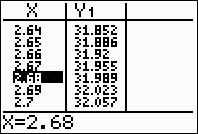The TABLE tells us that at 32º F there are 2.68 chirps per second.  So, what does this really mean?  Are the crickets cold? These findings are a bit deceiving.  At 32º F, the crickets are dead.  The lifespan of a cricket in a cold climate is very short.  The crickets spend the winter as eggs laid in the soil.  These eggs hatch in late spring or early summer, and tiny immature crickets called nymphs emerge.  Nymphs develop into adults within approximately 90 days. The adults mate and lay eggs in late summer before succumbing to old age or freezing temperatures in the fall. Also, remember that the further you extrapolate away from the data set, the less reliable the information will be.
.Finding Your Way Around TABLE of  CONTENTS